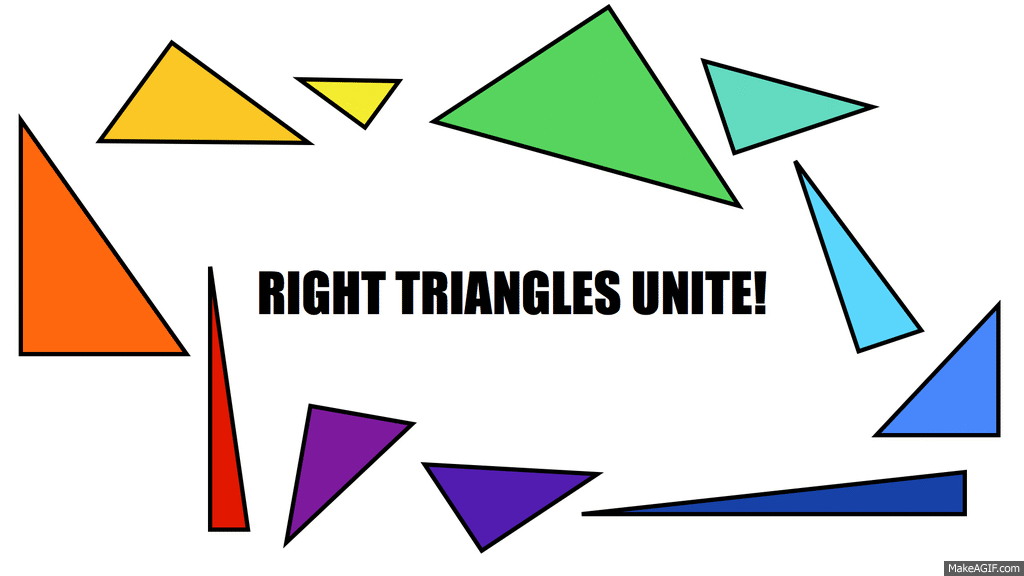The Pythagorean Theorem – an interactive lesson

This piece contains a mixture of reading and activities.  You can read the whole thing, or skim through for the activities.  Look for the word TASK to find the activities.

The Pythagorean Theorem.  It’s kind of a big deal.

Whether you know it or not, you probably rely on this theorem every day.  It defines a relationship between the three sides of a right triangle.  What’s a right triangle, you ask?  Here, let me introduce you:Just like beauty, right triangles come in many different shapes and sizes.TASK: Go play with the pile of triangles.  Separate the right triangles from the rest of the pile.

. . .

A right triangle will always have one side (called the hypotenuse) that’s longer than each of the other two sides (the legs).TASK:  Can you be sure the hypotenuse is always the longest side? Try to draw a right triangle where the hypotenuse is not the longest side.  What do you notice?  (Don’t have any paper?  Do your drawing here.)

. . .

The Pythagorean Theorem gives us the following relationship between the three sides.

Pythagorean Theorem:  in a right triangle, the sum of the squares of the two shorter lengths (the lengths of the legs) is equal to the square of the longer length (the length of the hypotenuse).The theorem enables you to solve problems like this one.TASK: Compute the distance in the picture above.

. . .

(scroll down for a solution)

. . .

SOLUTION:  Let’s call the ground distance x.  Since the 12-foot beam appears to be perpendicular to the ground (i.e. it makes an angle of 90 degrees with the ground), we can identify it as part of a right triangle.Now we use the Pythagorean Theorem.  The two legs of the triangle are 12 and x.  The hypotenuse is 15.Solve for x in the above equation:Since x2 = 81, we have x = 9 or x = -9.

So… it looks like we have two solutions.  Except one of them doesn’t make sense in the context of the problem.  Is it possible to have a distance of -9?  Not in this situation.

So x = 9 feet.

The ladder touches the ground 9 feet away from the tower.

What does this have to do with your life?

Right triangles are everywhere.  All you have to do is look around.  They were probably used to construct the building you’re in right now.   They could be as close as the chair you’re sitting on.

I found one under my desk.  Do you see it?I also found some right triangles outside my window.  I outlined one of them in pink.  Can you find the other ones?TASK:  Identify at least three more right triangles in the picture above.

TASK:  Identify the closest right triangle in your physical space.  Pay attention to furniture, ceilings, staircases, clothing, electronics, roofs, etc.  Note that the nearest right triangle may be behind you.

. . .

Ok.  Were you longing for something more majestic?

See if you can find any right triangles in the Golden Gate Bridge.Every time you see a right triangle in your world, either in a bridge, or the railing of a staircase, or a ramp, or the beams on the ceiling, it means someone somewhere was using the Pythagorean Theorem.

To practice applying this theorem to the triangles in your life, try these problems on Khan Academy:

Pythagorean Theorem – Practice

Pythagorean Theorem – Word Problems

Pythagorean Theorem – Challenge

Polygons, Bias, and Segregation

Here’s the latest from my internet idol, Vi Hart.

It’s an interactive post on math and game theory, that illustrates the effect of individual & collective bias in a culture. You could jump to the societal implications, or just have fun playing with the math and animations.

Great for use in a classroom or a casual setting.

It’s called Parable of the Polygons: http://ncase.me/polygons/